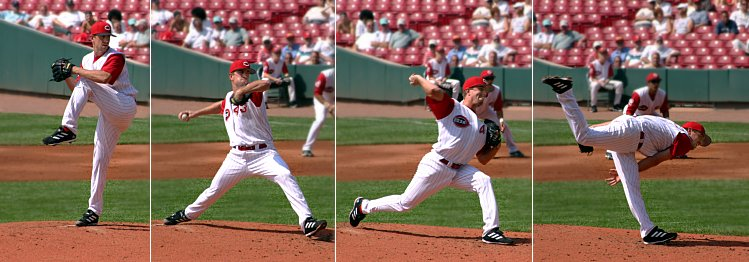# Joule

A joule is the SI base unit for energy. It is equal to . In physical terms, lifting an apple one meter takes 1 joule of energy. This should not be confused with a watt which is a unit of power and a rate of how fast energy is used.

While the joule is the SI base unit for energy, when speaking in real-world terms, we often use the kilowatt-hour (kWh) instead. This is due to the fact that a joule is an extremely small amount of energy. To put how small a joule is into perspective, a liter of gasoline has 31,536,000 joules of energy in it. A kilowatt-hour is equal to 3,600,000 joules. Therefore, a liter of gasoline has 8.76 kW/hr of energy in it, which is a much more manageable number.Figure 1. A 250 g baseball traveling at 20 m/s (72 kph) has 50 joules of kinetic energy.